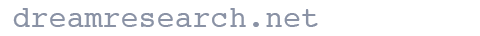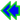To find an h score from two percentages, simply look up both percentages in this table and find the difference between their corresponding X's. For instance, scores of 50% and 25% yield an h of 1.571 - 1.047 = .524.

%X
00.000
10.200
20.284
30.348
40.403
50.451
60.495
70.536
80.574
90.609
100.644
110.676
120.707
130.738
140.767
150.795
160.823
170.850
180.876
190.902
200.927
210.952
220.976
231.000
241.024
%X
251.047
261.070
271.093
281.115
291.137
301.159
311.181
321.203
331.224
341.245
351.266
361.287
371.308
381.328
391.349
401.369
411.390
421.410
431.430
441.451
451.471
461.491
471.511
481.531
491.551
%X
501.571
511.591
521.611
531.631
541.651
551.671
561.691
571.711
581.731
591.752
601.772
611.793
621.813
631.834
641.855
651.875
661.897
671.918
681.939
691.961
701.982
712.004
722.026
732.049
742.071
%X
752.094
762.118
772.141
782.165
792.190
802.214
812.240
822.265
832.292
842.319
852.346
862.375
872.404
882.434
892.465
902.498
912.532
922.568
932.606
942.647
952.691
962.739
972.793
982.858
992.941

You can also find X and h by using a mathematical formula. The formula is: X is the anticosine (using radians) of 1 - 2P, where P is a number from 0 to 1 (not 0 to 100). h is simply the difference between the two X's:

h = cos-1(1-2Pa) - cos-1(1-2Pb)

In Excel, assuming the two proportions are in cells A2 & B2, the formula would be: =ACOS(1-(2*A2))-ACOS(1-(2*B2))Go back to the Statistics page.